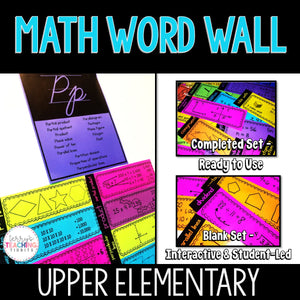# Math Word Wall {Upper Elementary}

• \$6.00
Unit price per
Shipping calculated at checkout.

This resource contains various options for creating a word wall in your upper elementary math classroom - it can even become interactive and student-led.

Vocabulary words included
Algebra, Algorithm , Array, Addition, Add, Acute angle, Acute triangle, Attribute, Area of base, Axis, Axes, Associative property of multiplication, Base, Benchmark fraction, Common denominator, Congruent, Conversion, Convert, Cubic units, Coordinates, Coordinate plane, Customary system, Customary unit of measure, Commutative property of multiplication, Distributive property, Dividend, Decimal, Decimal point, Division, Divisor, Difference, Denominator, Equation, Exponent, Expression, Equivalent, Estimate, Equilateral triangle, Edge lengths, Fraction, Factor, First quadrant, Gap, Hundredths, Hundred thousands, Hundreds, Hexagon, Height, Horizontal, Identity property of multiplication, Improper fraction, Irregular polygon, Isosceles triangle, Intersection of lines, Kite, Line plot, Length, Liquid volume, Line, Multiplicand, Multiplier, Millions, Multiple, Mixed number, Measurement, Metric system, Mass, Multiplicand, Multiplier, Millions, Multiple, Mixed number, Measurement, Metric system, Mass, Metric unit of measure, Numerator, Order of operations, Ones , Obtuse angle, Ordered pairs, Origin, Partial product, Partial quotient, Product, Place value, Power of ten, Parallel lines, Parallelogram, Pentagon, Plane figure, Polygon, Point, Partition division , Properties of operations, Perpendicular lines, Quotient , Quadrilateral, Repeated subtraction, Remainder, Rounding , Reasonableness, Rectangle, Regular polygon, Right angle, Right triangle, Rhombus/rhombi, Rule, Right rectangular prism, Simplify, Subtraction, Subtract, Scalene triangle, Square, Solid figure, Tenths, Thousandths, Ten-thousands, Tens, Thousands, Triangle, Trapezoid, Two-dimensional, Unlike denominator, Unit fraction, Unit, Unit cube, Vertex, Volume, Vertical, Weight, Width, x-axis, x-coordinate, y-axis, y-coordinate